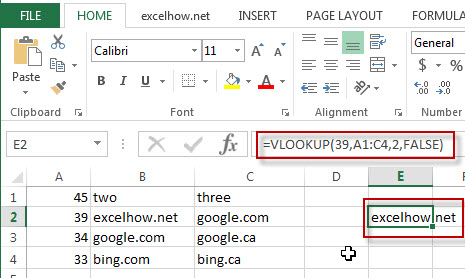# Excel Vlookup Function

This post will guide you how to use Excel VLOOKUP function with syntax and examples in Microsoft excel.

## Description

The Excel VLOOKUP function lookup a value in the first column of the table and return the value in the same row based on index_num position.

The VLOOKUP function is a build-in function in Microsoft Excel and it is categorized as a Lookup and Reference Function.

The VLOOKUP function is available in Excel 2016, Excel 2013, Excel 2010, Excel 2007, Excel 2003, Excel XP, Excel 2000, Excel 2011 for Mac.

## Syntax

The syntax of the VLOOKUP function is as below:

`= VLOOKUP (lookup_value, table_array, column_index_num,[range_lookup])`

Where the VLOOKUP function arguments are:

• Lookup_value -This is a required argument. The value that you want to search for, in the first column of the table. Note: the lookup_value can be a value, a cell or ranges reference, or a text string.
• Table_array – This is a required argument. Two or more columns of data to be searched in the first column.
• column_index_num – This is a required argument.  The column number in table_array.
• Range_lookup – This is a optional argument.  The value can be set as True or False. If true, the function will return an approximate match value. If False, it will find an exact match or it will return an error value #N/A.

## Example

The below examples will show you how to use Excel VLOOKUP Lookup and Reference Function.

#1 To search “39” text string in the first column and returns the value from column 2 that is in the same row, just using the following excel formula: =VLOOKUP(39,A1:C4,2,FALSE)### Related Formulas

• Check If a Value Exists in a Range
This post will guide you how to use VLOOKUP function to check if a value exists in a given range of cells in Excel. How to check if a specified value exists in a range and then return the value in the adjacent cell…
• VLOOKUP Values from Multiple Worksheets
you can use the VLOOKUP function to find the values from multiple worksheets in Excel. For example, assuming that you have two worksheets, and you want to search the data across those two worksheet in your current worksheet (sheet3)……
• Excel Vlookup Return True or False
you can use the VLOOKUP function to look for a value in a column in a table and then returns TRUE from a given column in that table if it finds something. If it doesn’t, it returns FALSE …
• VLOOKUP Return Multiple Values Horizontally
You can create a complex array formula based on the INDEX function, the SMALL function, the IF function, the ROW function and the COLUMN function to vlookup a value and then return multiple corresponding values horizontally in Excel.…